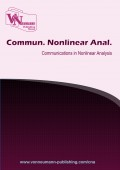# An Existence Result For A Class Of Nonlinear Volterra Functional Integral Equations

Volume 1, Issue 1, pp 1-7### Authors

Neda Khodabakhshi - Department of Mathematics and Computer Science, Amirkabir University of Technology, Tehran, Iran
Adrian Petrusel - bFaculty of Mathematics and Computer Science, Babe-Bolyai University Cluj-Napoca, Koglniceanu Street, No. 1, Cluj-Napoca, Romania.

### Abstract

Recently [N. Khodabakhshi, S. M. Vaezpour, Fixed Point Theory, to appear] provides sufficient conditions for the existence of common fixed point for two commuting operators using the technique associated to an abstract measure of non-compactness in Banach spaces. In this paper, we develope their work with further applicative investigation. More precisely, we give suitable assumptions in order to obtain the existence of solutions for a nonlinear integral equation.

### Keywords

Coincidence point, common fixed point, measure of noncompactness

### References

 R. P. Agarwal, M. Meehan, D. O’Regan, Fixed Point Theory and Applications, Cambridge University Press, Cambridge, (2004). 2
 A. Aghajani, J. Bana´s, N. Sabzali, Some generalizations of Darbo fixed point theorem and applications, Bull. Belg. Math. Soc. Simon Stevin., 20 (2013), 345–358. 1
 A. Aghajani, Y. Jalilian, Existence and global attractivity of solutions of a nonlinear functional integral equation, Commun. Nonlinear Sci. Numer. Simul., 15 (2010), 3306–3312. 1
 J. Bana´s, On measures of noncompactness in Banach spaces, Comment. Math. Univ. Carolinae., 21 (1980), 131–143. 1
 J. Bana´s, Measures of noncompactness in the space of continuous tempered functions, Demonstratio Math., 14 (1981), 127–33. 3
 J. Bana´s, B. C. Dhage, Global asymptotic stability of solutions of a functional integral equation, Nonlinear Anal. 69 (2008), 1945–1952. 1
 J. Bana´s, K. Goebel, Measures of Noncompactness in Banach spaces, Lect. Notes Pure Appl. Math., vol. 60, Dekker, New York, (1980). 2, 2
 J. Bana´s, B. Rzepka, An application of a measure of noncompactness in the study of asymptotic stability, Appl. Math. Lett., 16 (2003), 1–6. 1
 J. Dugundji, A. Granas, Fixed Point Theory, vol. 1, PWN, Warszawa, (1982). 2.4
 W. G. El-Sayed, Nonlinear functional integral equations of convolution type. Port. Math., 54 (1997), 449–456
 A. Hajji, E. Hanebaly, Commuting mappings and α-compact type fixed point theorems in locally convex spaces, Int. J. Math. Anal., 1 (2007), 661–680. 1
 A. Hajji, A generalization of Darbo’s fixed point and common solutions of equations in Banach spaces, Fixed Point Theory Appl., 2013 (2013), 9 pages .
 X. Hu, J. Yan, The global attractivity and asymptotic stability of solution of a nonlinear integral equation, J. Math. Anal. Appl., 321 (2006), 147–156
 N. Khodabakhshi, S. M. Vaezpour, Common fixed point theorems via measure of noncompactness, Fixed Point Theory, to appear.
 Z. Liu, S. Kang, J. Ume, Solvability and asymptotic stability of an nonlinear functional-integral equation, Appl. Math. Lett., 24 (2011), 911–917
 Y. C. Wong, Introductory Theory of Topological Vector Spaces, Marcel Dekker, Inc., New York, (1992